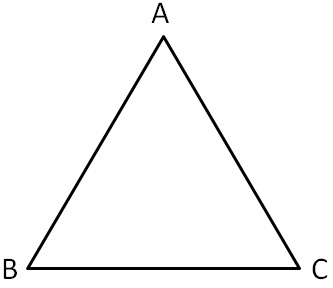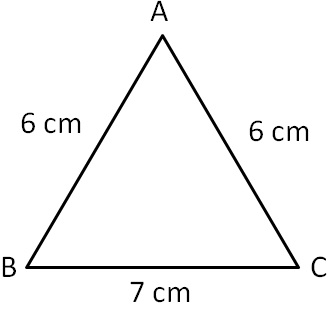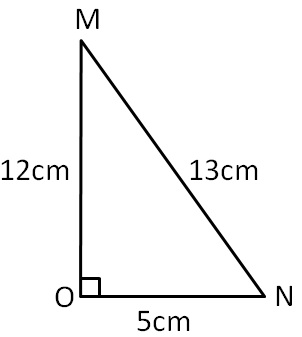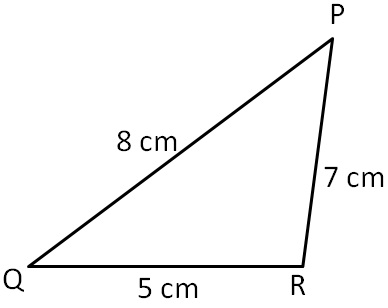Sum of lengths of two sides of a triangle

Chapter 6 Class 7 Triangle and its Properties
Concept wise

In a triangle,

Sum of two sides > Third side.

In a triangle,

Sum of two sides is always greater than the third side.

For ∆ABCHere,

Sum of any sides is greater than the third side

AB + AC > BC

AB + BC > AC

BC + AC > AB

Let’s take a few examples

## Show that sum of any two sides is greater than the third side

In ∆ABC,

AB = 6 cm

BC = 7 cm

CA = 6 cmTwo sides Sum of two sides Third side Greater AB, BC 6 + 7  = 13 cm CA = 6 cm Yes BC, CA 7 + 6 = 13 cm AB = 6 cm Yes AB, CA 6 + 6 = 12 cm BC = 7 cm Yes

Since sum of any two sides is greater than the third side.

∴ They form a triangle

## Show that sum of any two sides is greater than the third side

In ∆MON,

MN = 13 cm

ON = 5 cm

OM = 12 cmTwo sides Sum of two sides Third side Greater MN, ON 13 + 5  = 18 cm OM = 12 cm Yes ON, OM 5 + 12 = 17 cm MN = 13 cm Yes MN, OM 13 + 12 = 25 cm ON = 5 cm Yes

Since sum of any two sides is greater than the third side.

∴ They form a triangle

## Show that sum of any two sides is greater than the third side

In ∆PQR,

PQ = 8 cm

QR = 5 cm

PR = 7 cmTwo sides Sum of two sides Third side Greater PQ, QR 8 + 5  = 13 cm PR = 7 cm Yes QR, PR 5 + 7 = 12 cm PQ = 6 cm Yes PR, PQ 8 + 7= 15 cm QR = 5 cm Yes

Since sum of any two sides is greater than the third side.

∴ They form a triangle

Learn in your speed, with individual attention - Teachoo Maths 1-on-1 Class